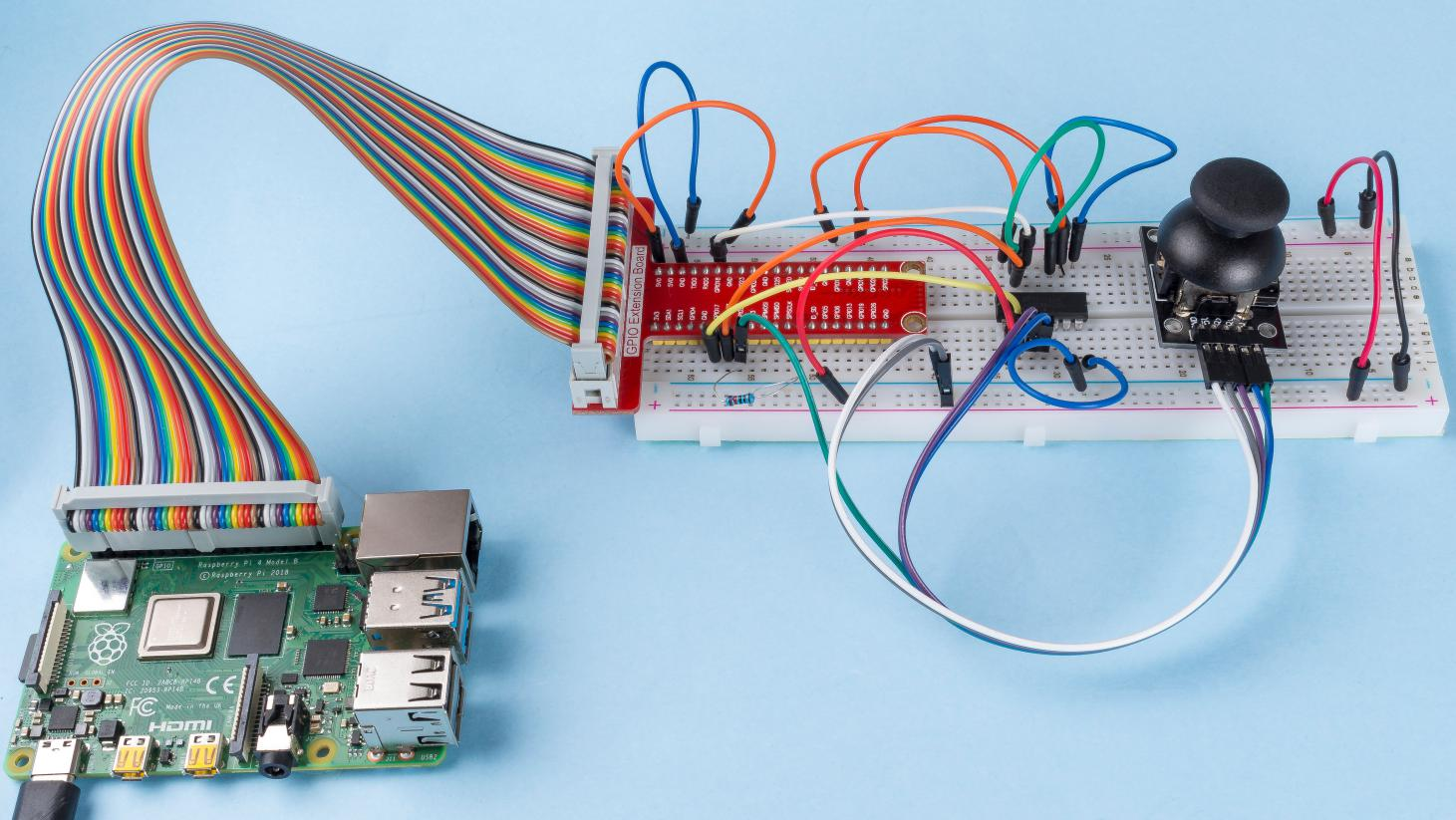# 2.1.9 Joystick¶

## Introduction¶

In this project, We’re going to learn how joystick works. We manipulate the Joystick and display the results on the screen.

## Components¶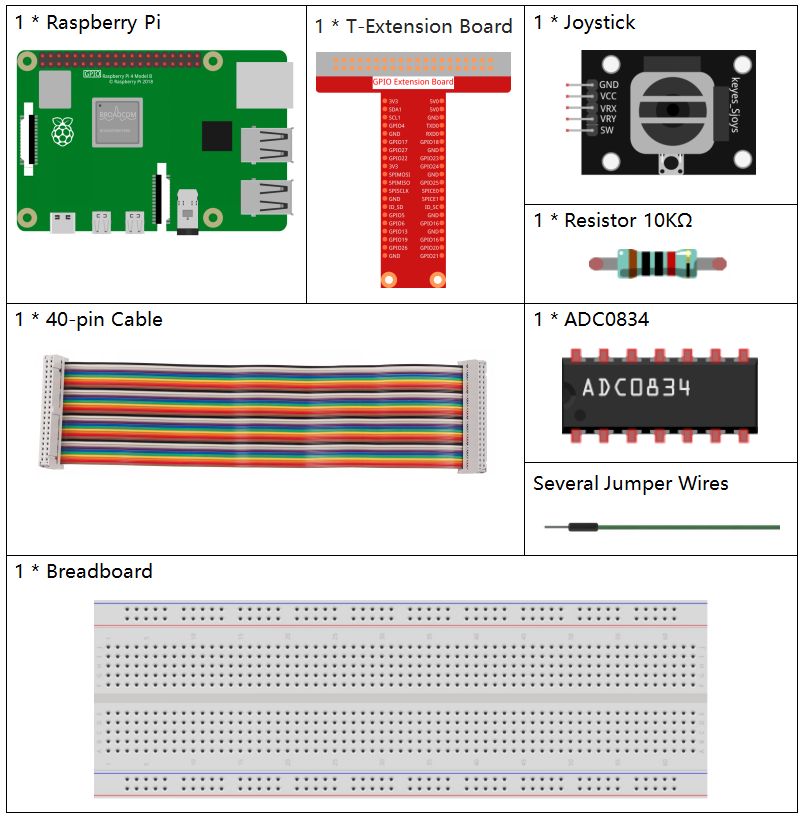## Schematic Diagram¶

When the data of joystick is read, there are some differents between axis: data of X and Y axis is analog, which need to use ADC0834 to convert the analog value to digital value. Data of Z axis is digital, so you can directly use the GPIO to read, or you can also use ADC to read.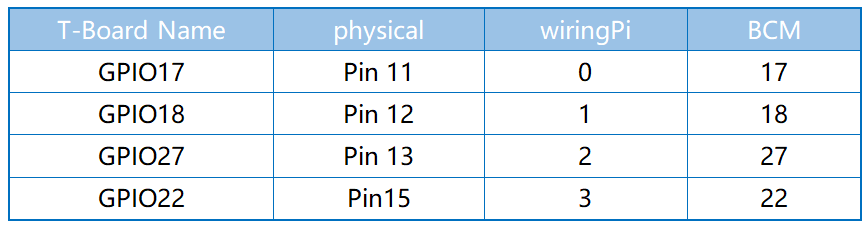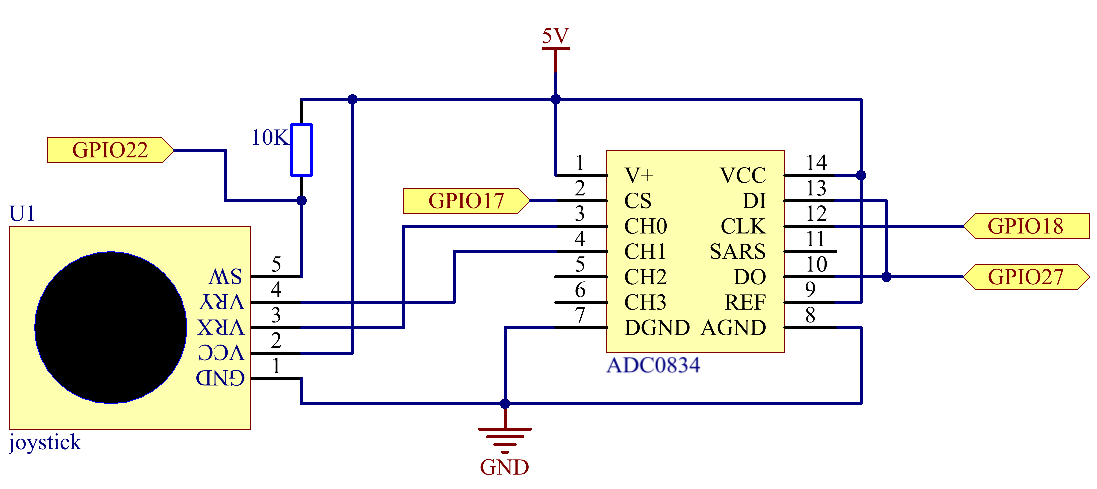## Experimental Procedures¶

Step 1: Build the circuit.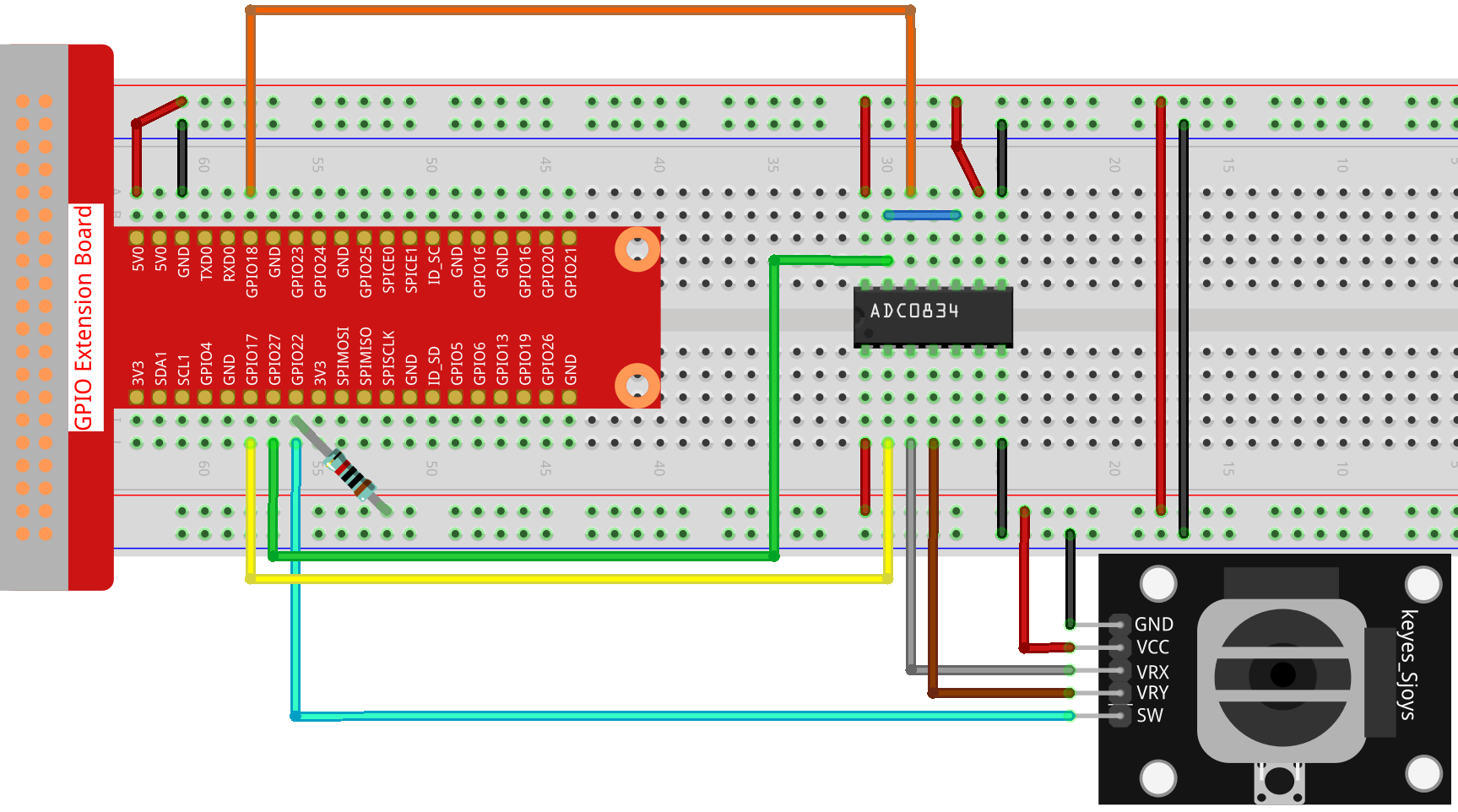Step 2: Go to the folder of the code.

```cd /home/pi/raphael-kit/nodejs/
```

Step 3: Run the code.

```sudo node joystick.js
```

After the code runs, turn the Joystick, then the corresponding values of x, y, Btn are displayed on screen.

Code

```const Gpio = require('pigpio').Gpio;

const btn = new Gpio(25, {
mode: Gpio.INPUT,
pullUpDown: Gpio.PUD_UP,
});

setInterval(async() => {

console.log(`x = \${x_val}, y = \${y_val}, btn = \${btn_val}\n`);
}, 100);
```

Code Explanation

```const ADC0834 = require('./adc0834.js').ADC0834;
```

We import an `ADC0834` constructor to use the adc0834 module.

```setInterval(async() => {